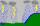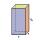# Annual rainfall

The average annual rainfall is 686 mm. How many liters will fall on the 1-hectare field?

Result

V =  6860000 l

#### Solution:Leave us a comment of example and its solution (i.e. if it is still somewhat unclear...):

Showing 0 comments:Be the first to comment!#### To solve this example are needed these knowledge from mathematics:

Do you want to convert area units? Do you want to convert length units? Do you know the volume and unit volume, and want to convert volume units?

## Next similar examples:

1. RainfallOn Thursday, fell 1 cm rainfall. How many liters of water fell to rectangular garden with dimensions of 22 m x 35 m?
2. RainfallHow many liters of water did fell in a 32m long and 8m wide garden, if 8mm of rain fell?
3. Water reservoirThe water tank has a cuboid with edges a= 1 m, b=2 m , c = 1 m. Calculate how many centimeters of water level falls, if we fill fifteen 12 liters cans.
4. Cylindrical tank 2If a cylindrical tank with volume is used 12320cm raised to the power of 3 and base 28cm is used to store water. How many liters of water can it hold?
5. The shopThe shop has 3 hectoliters of water. How many liter bottles is it?
6. One hectareHow many square meters are one hectare?
7. Percitipation - meteoDo you have any tip for calc percitipation on a lnd ? Example on 2 ha falls to 5 mm, how many cubic meters is it?
8. Argicultural fieldField has a rectangular shape with a width 180 m and circumference 940 m. How many hectares acreage has field?
9. HectolitersHow many hectoliters of water fits into cuboid tank with dimensions of a = 3.5 m b = 2.5 m c = 1.4 m?
10. Concrete pillarHow many m³ of concrete is needed for the construction of the pillar shape of a regular tetrahedral prism, when a = 60 cm and the height of the pillar is 2 meters?
11. Bathroom 2A bathroom is 2.4 meters long and 1.8 meters wide. How many square tiles 1 dm on each side are to be used to cover it?
12. Pool in litresPool has a width of 3.5 m length of 6 m and a height 1.60 meters. Calculate pool volume in liters.
13. The tankThe tank has 1320 liters of water. The tank has the shape of a prism, its base is an rectangle with sides a = 0,6 m and b = 1,5 m. How high does the water level reach in the tank?
14. RapeThe agricultural cooperative harvested 525 ares of rape, of which received 5.6 tons of rape seeds. Calculate the yield per hectare of rape.
15. LandLand has a rectangular shape, its surface area is 1.45 hectares. Its width is 250 m. Determine the length of the land.
16. Customary lengthConvert length 65yd 2 ft to ft
17. SandboxSandbox has area of 32 sq ft and length of 4 1/2 ft. What is width of sandbox.